# Data augmentation

Is a neural network more robust thanks to data augmentation?

## Saimple : Robustness of Data Augmentation

Saimple allows us to measure the robustness of a neural network thanks to a criteria named delta max. The delta max corresponds to the delta above which dominance can no longer be guaranteed. This delta, in the case of a classifier for example, acts on the level of perturbations added to the input image. The higher the delta value, the more disturbances there are.

## 1. Definitions

• Robustness defines the degree to which a system maintains correct behaviour despite the presence of perturbations.
• A proof of dominance of a class corresponds to the fact that on the space considered the response of the neural network on this class is strictly superior to the responses of all the other classes.
• The delta max corresponds to the largest radius of the N-norm hyperball on which it is possible to prove dominance. In the exact case, the delta max corresponds to the distance to the N-norm of the nearest adversary example.
• A hyperball of radius d to norm N, corresponds to a volume of dimension d, including the set of points located at a distance less than or equal to d of norm N from the centre of the hyperball.
• The larger the hyperball, the more points it contains. If one of the points included in this hyperball is an adversary example, then it is no longer possible to prove dominance. Thus, the delta max corresponds to the largest hyperball that only includes points of the same class.
• The larger the delta max, the more robust the network is in the region considered.
• Loss: loss function which represents the difference between the predictions made by the neural network and the real values of the observations. The closer it is to 0, the better.
• Accuracy: percentage of success of predictions, i.e. the prediction corresponds to the real value.

## 2. Presentation of the Use case

In this use case we use a classifier (see architecture in appendices) trained on the SignLanguageDigits dataset.

The model named "Original" is the neural network trained on the non-augmented dataset. The model named "Augmented" is the neural network trained on the augmented dataset, where the brightness of the images has been modified.

The dataset is composed of 10 classes. Each of these classes represents the value of a number (ranging from 0 to 9) in sign language. Thus, the sign 0 will be represented by the output class 0 and so on.

To bring data augmentation into play, we increased the size of the dataset by a factor of 3 by varying the brightness of the training images. However, the validation and test images were not modified.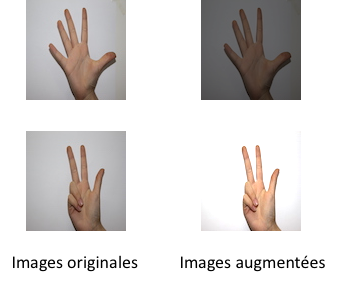Example of original image and after augmentation

The aim of this study is to check whether a model with better accuracy and loss values is more robust. As the two models below have a close accuracy, it is difficult to distinguish which is better based on this data alone.

 Models Loss Accuracy Original 0.1200 0.9878 Augmented 0.0704 0.999

There is less loss in the model with the augmented data set. The accuracy of the augmented model is better but still close to the original model.

## 3. Experiments

To perform our experiments we took a sample of 10 images (each representing a class).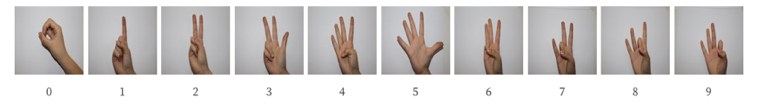Illustration of the 10 reference classes

To illustrate the behaviour of the models, we use a radar with data from the dominance results of the Saimple evaluations.

A curve is composed of 10 points (10 points, as 10 output classes). One point corresponds to the classification score of a class. The class with the highest score will be the class selected by the model.

For the purpose of simplified visualisation, the classification score displayed on the radar corresponds to the maximum value that the neural network can respond to in the space of the inputs considered. The colours of the curves are chosen randomly from one radar to another.

The centre of the radar corresponds to the value 0 and its periphery the value 1.

Two examples of evaluations with a picture representing a 3 sign :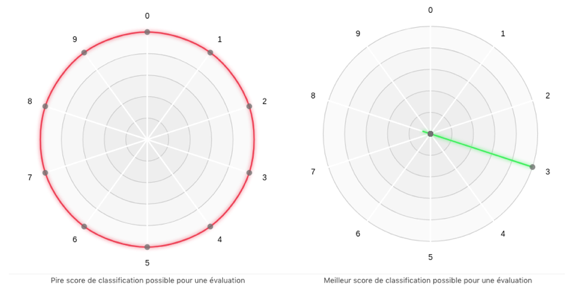In the worst case, no class is dominant, they are all in conflict. In the best case, class 3 is dominant with a score of 1 and the other 9 have a score of 0.

### 3.1 Dominance radar at delta 0

The first experiment performed with Saimple compares the scores of our two models with a delta equal to 0 (a delta of 0 corresponds to a concrete evaluation, i.e. without noise).

On the left the dominance radar with the "Original" model. On the right the dominance radar with the "Augmented" model. These 2 dominance radars contain 10 evaluations. Each one is performed on one of the 10 reference images.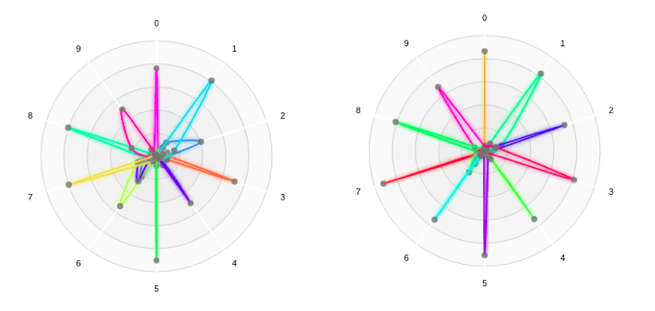Original                                                                             Augmented

Note: Each curve is drawn from a dominance result of an evaluation with a model and one of the 10 images presented above.

The model on the left is less accurate. The curves are less close to the periphery and more close to the centre. Whereas the model on the right illustrates dominances that are well demarcated for each of the figures. We can therefore understand from the analysis carried out with Saimple that the augmented model is much more efficient.

### 3.2 Dominance radar at delta max

The second experiment consisted in looking for the delta max for each of the 10 reference images on the 2 models.

Below, the scores of our 2 models with their respective delta max on the 10 reference images.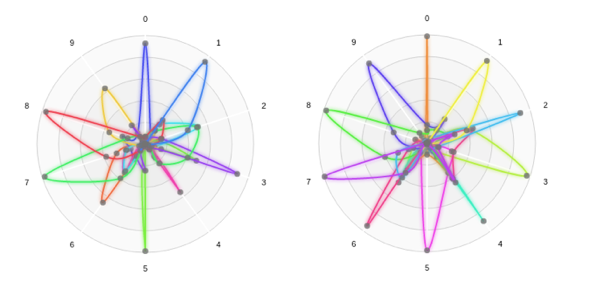Original                                                              Augmented

Note: Each curve is derived from a dominance result of an evaluation with a model and one of the 10 images presented earlier.

Graph of max deltas by class and model :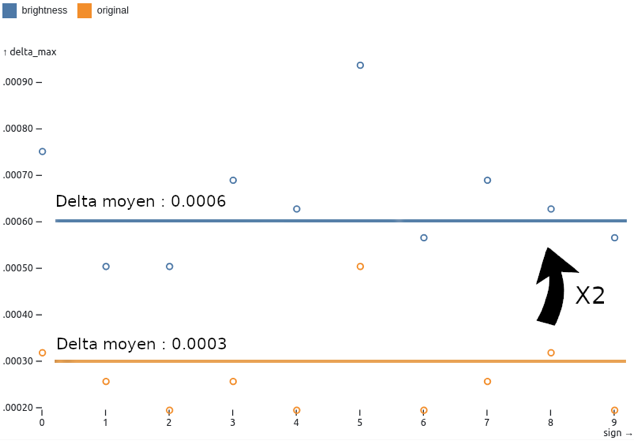Reminder: The higher the delta max value, the more robust the model is

It can be seen from the evaluation that the values of the max deltas of the "Augmented" model are always higher than those of the "Original" model. Thus the model has been made more robust by the data augmentation.

By averaging the ratios of the max deltas over 10 evaluations between the two models, we notice that the max delta of the "Augmented" model is twice as large as that of the "Original" model.

### 3.3 Dominance radar at delta max for specific evaluations

With Saimple, the delta max is obtained by dichotomous search. For this sample of 10 images, the search was oriented in the interval [0.00001: 0.001]. Each dominance radar below corresponds to an individual evaluation for a number in the delta max for a model and an image.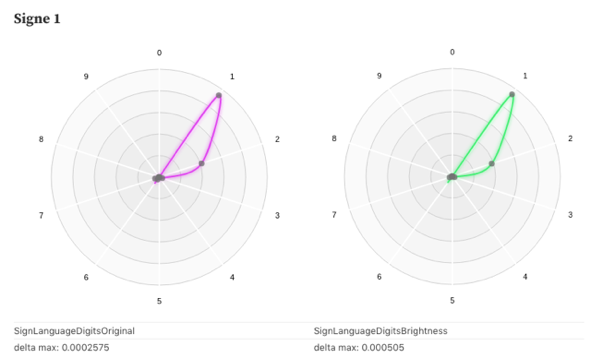Above is an example where data augmentation has not significantly changed the classification scores.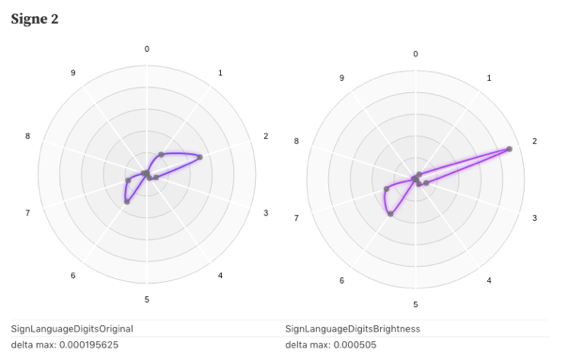In this example, the data augmentation has greatly improved the delta max. Class 2 is much more dominant.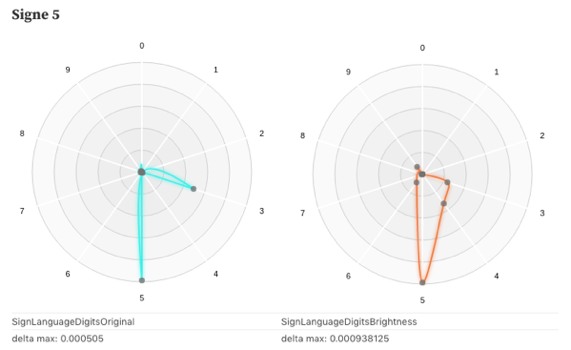Finally, in this example the data augmentation decreased the score for class 3 but increased the score for class 4.

With Saimple we can therefore clearly identify the impacts of data augmentation on datasets and visualise how this affects model performance. Even if the overall performance is improved, there may be some elements where nothing has changed, or on the contrary some where the performance may deteriorate, as shown above.

However, in most of the radars with the Augmented model, the curves are more stretched towards the reference class. This implies that the overall robustness has been improved.

## 4. Conclusion

The delta max ratio gives us a good idea of the difference in robustness between the two models. The delta max of the "Augmented" model is on average 2 times larger than the "Original" model. The dominance radars also allow us to better visualise the behaviour of the model when faced with their delta max. Saimple therefore allows us to verify on a sample of images that the "Augmented" model is more robust, while showing the impact of the data augmentation on this model.

If you are interested in Saimple, want to know more about the use case or if you want to have access to a demo environment of Saimple: